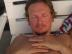## First p2 milestone passed: parse 50% of perl5

This week I spent some time to fix the remaining p2 parser problems. I set my first goal to parse 50% of perl5 syntax by summer 2013. I want to show some real code and benchmarks at the upcoming YAPC::Asia in Tokyo.

After the YAPC::EU in Kiev I first spent some time fixing B::C for 5.16, 5.18 and blead (PADLIST, COW and bytecode compiler regressions). In the meantime my partner in crime goccy in Tokyo made progress with his parser, compiler and the new llvm backend. His parser is hand-written and I'm still fighting with greg, but my code is still much smaller and more elegant.

I spent most of the last days fixing the expression parser and how to parse functions and method calls. Every expr is a list (TUP for tuple), and calls can be like `call arg1, args...` (implicit list context), `call call args...` (nested calls), `call(list)` (explicit lists), and similar for methods. Add indirect method calls to the mix that p2 does only methods, no functions calls. Every call is a method one some object, user-provided subs not in a class (or package) are stored globally and act on the global 'Lobby' object.

``````expr = c:method           { \$\$ = PN_AST(EXPR, c) }
| c:calllist          { \$\$ = PN_AST(EXPR, c) }
| c:call e:expr       { \$\$ = PN_AST(EXPR, PN_PUSH(PN_S(e,0), PN_S(c,0))); }
| c:call l:listexprs  { \$\$ = PN_SHIFT(PN_S(l,0));
if (!PN_S(l, 0)) { PN_SRC(c)->a = PN_SRC(\$\$); }
\$\$ = PN_PUSH(PN_TUP(\$\$), c); }
| e:opexpr            { \$\$ = PN_AST(EXPR, PN_TUPIF(e)) }
| c:call              { \$\$ = PN_AST(EXPR, c) }
| e:atom              { \$\$ = PN_AST(EXPR, PN_TUPIF(e)) }

calllist = m:name - list-start - list-end
{ PN_SRC(m)->a = PN_SRC(PN_AST(LIST, PN_NIL)); \$\$ = PN_TUP(m) }
| m:name - l:list -
{ PN_SRC(m)->a = PN_SRC(l); \$\$ = PN_TUP(m) }
| m:name - list-start l:callexprs list-end -
{ PN_SRC(m)->a = PN_SRC(PN_AST(LIST, l)); \$\$ = PN_TUP(m) }
call = m:name - { \$\$ = PN_TUP(m) }
method = v:methlhs - arrow m:name - l:list -
{ PN_SRC(m)->a = PN_SRC(l); \$\$ = PN_PUSH(PN_TUPIF(v), m) }
| v:methlhs - arrow m:name -
{ \$\$ = PN_PUSH(PN_TUPIF(v), m) }
``````

`methlhs` is a name or \$scalar. The biggest problem was some missing whitespace, and the differences of signature parsing, from the weird potion way, which compiles expr in the compiler to sigs to the new way in p2, where the parser already generates proper signatures. The `PN_SHIFT(PN_S(l,0))` orgy above in `call listexprs` is for moving the object from the first arg of the call to the front, the indirect method call. I'm not happy with that.

All my perl5 tests pass now, which means there are a lot of new features to explore, like declaring default parameters and calling with named parameters (no need for hash abuse anymore).

``````\$ cat test/closures/named.pl
sub min (\$x, \$y) { \$y - \$x }
@b = (99, 98, 97);
\$b = "XXX";
(1, min(\$y=12, \$x=89), \$b, \$b) #=> (1, -77, 97, XXX)

\$ cat test/closures/default.pl
sub min (\$x=0, \$y=1) { \$y - \$x }
(min(), min(1), min(0,1), min(\$y=0), min, min->arity, min->minargs)
#=> (1, 0, 1, 1, sub(\$x:=0,\$y:=1), 2, 0)
``````

And this is the p2 parse tree

``````\$ bin/p2 -Dv test/closures/default.pl

-- parsed --
code (assign (expr (msg ("min")) expr (proto (list (\$x, 58, 0, \$y, 58, 1) block (expr (minus (msg ("\$y") msg ("\$x"))))))), expr (list (expr (msg ("min" list undef undef)), expr (msg ("min" list (expr (value (1))) undef)), expr (msg ("min" list (expr (value (0)), expr (value (1))) undef)), expr (msg ("min" list (assign (expr (msg ("\$y")) expr (value (0)))) undef)), expr (msg ("min")), expr (msg ("min"), msg ("arity")), expr (msg ("min"), msg ("minargs")))))
``````

The sig `(\$x=0, \$y=1)` is parsed to `(\$x, 58, 0, \$y, 58, 1)`, `58` being `chr(:)`. potion uses the `=` for type assignment and `:=` for defaults, hence `:` instead of `=`. The 3rd element for each sig, here 0 denotes the default value, which can only be immediate values for now. It could be an expression also, but I dislike the idea. Looks like action at a distance.

Currently all variables and subs are lexical only, work for dynamic symbol lookup is still in a branch. And no example from the shootout benchmark works yet, as I haven't implemented yet for loops, recursive function calls are buggy and similar stuff.

Since the underlying parse tree and vm code is 1:1 the same as for potion, the benchmarks are the same as for potion, i.e. typically 30x faster than perl5 code.Working at cPanel on cperl, B::C (the perl-compiler), parrot, B::Generate, cygwin perl and more guts, keeping the system alive.